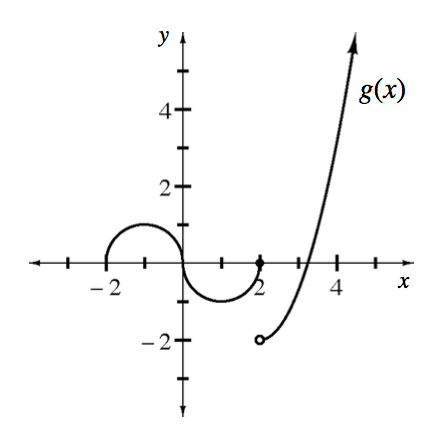### Home > APCALC > Chapter 8 > Lesson 8.2.2 > Problem8-86

8-86.

Multiple Choice: The graph of $y = g\left(x\right)$ shown below has horizontal tangents at $\left(–1, 1\right)$ and $\left(1, –1\right)$. It has vertical tangent at $\left(0, 0\right)$. For what values of $x$ on the open interval $\left(–2, 4\right)$ is $g$ not differentiable?

1. $2$ only

1. $0$ and $2$

1. $−1$ and $1$

1. $0$ only

1. $−1, 0, 1$, and $2$First of all, differentiability implies continuity. (That is, a point of discontinuity does not have a derivative.) Secondly, the slopes must agree from the left and the right.

$\left (\text{That is to say, }\lim \limits_{x\rightarrow a^{-}}g'(x)=\lim \limits_{x\rightarrow a^{+}}g'(x) \right )$

Next, the value of the derivative must exist. (That is, the derivative must be finite.) Lastly, the value of the derivative must agree with the limit of the derivative at that point.